# JP5786571B2 - Power semiconductor device temperature measurement device - Google Patents

## Info

Publication number
JP5786571B2
JP5786571B2 JP2011194942A JP2011194942A JP5786571B2 JP 5786571 B2 JP5786571 B2 JP 5786571B2 JP 2011194942 A JP2011194942 A JP 2011194942A JP 2011194942 A JP2011194942 A JP 2011194942A JP 5786571 B2 JP5786571 B2 JP 5786571B2
Authority
JP
Japan
Prior art keywords
temperature
value
temperature detection
converter
output
Prior art date
Legal status (The legal status is an assumption and is not a legal conclusion. Google has not performed a legal analysis and makes no representation as to the accuracy of the status listed.)
Active
Application number
JP2011194942A
Other languages
Japanese (ja)
Other versions
JP2013057550A (en
Inventor

Original Assignee

Priority date (The priority date is an assumption and is not a legal conclusion. Google has not performed a legal analysis and makes no representation as to the accuracy of the date listed.)
Filing date
Publication date
Application filed by 富士電機株式会社 filed Critical 富士電機株式会社
Priority to JP2011194942A priority Critical patent/JP5786571B2/en
Publication of JP2013057550A publication Critical patent/JP2013057550A/en
Application granted granted Critical
Publication of JP5786571B2 publication Critical patent/JP5786571B2/en
Application status is Active legal-status Critical
Anticipated expiration legal-status Critical

## Images

•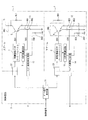•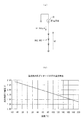•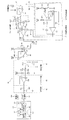••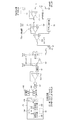•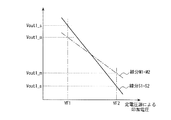••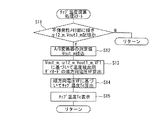•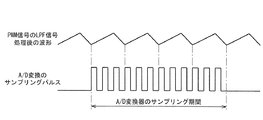•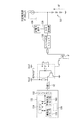•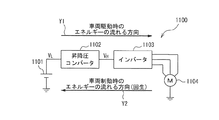•••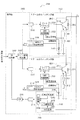•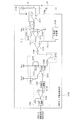•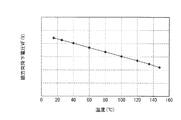•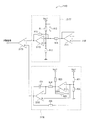•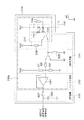••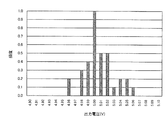••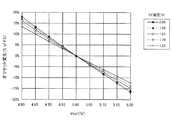••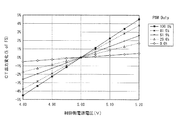## Classifications

• GPHYSICS
• G01MEASURING; TESTING
• G01KMEASURING TEMPERATURE; MEASURING QUANTITY OF HEAT; THERMALLY-SENSITIVE ELEMENTS NOT OTHERWISE PROVIDED FOR
• G01K3/00Thermometers giving results other than momentary value of temperature
• G01K3/08Thermometers giving results other than momentary value of temperature giving differences of values; giving differentiated values
• G01K3/14Thermometers giving results other than momentary value of temperature giving differences of values; giving differentiated values in respect of space
• GPHYSICS
• G01MEASURING; TESTING
• G01KMEASURING TEMPERATURE; MEASURING QUANTITY OF HEAT; THERMALLY-SENSITIVE ELEMENTS NOT OTHERWISE PROVIDED FOR
• G01K15/00Testing or calibrating of thermometers
• G01K15/005Calibration
• GPHYSICS
• G01MEASURING; TESTING
• G01KMEASURING TEMPERATURE; MEASURING QUANTITY OF HEAT; THERMALLY-SENSITIVE ELEMENTS NOT OTHERWISE PROVIDED FOR
• G01K7/00Measuring temperature based on the use of electric or magnetic elements directly sensitive to heat ; Power supply, e.g. by thermoelectric elements
• G01K7/01Measuring temperature based on the use of electric or magnetic elements directly sensitive to heat ; Power supply, e.g. by thermoelectric elements using semiconducting elements having PN junctions
• GPHYSICS
• G01MEASURING; TESTING
• G01KMEASURING TEMPERATURE; MEASURING QUANTITY OF HEAT; THERMALLY-SENSITIVE ELEMENTS NOT OTHERWISE PROVIDED FOR
• G01K2219/00Thermometers with dedicated analog to digital converters
• HELECTRICITY
• H03BASIC ELECTRONIC CIRCUITRY
• H03KPULSE TECHNIQUE
• H03K17/00Electronic switching or gating, i.e. not by contact-making or -braking
• H03K17/08Modifications for protecting switching circuit against overcurrent or overvoltage
• H03K2017/0806Modifications for protecting switching circuit against overcurrent or overvoltage against excessive temperature

## Description

The present invention relates to a temperature measuring device for a power semiconductor device incorporated in a voltage converter device for a vehicle or the like.

In recent years, in vehicle equipment, as a measure for improving efficiency and saving energy, a vehicle drive system 1100 having an electric motor 1104 that generates a driving force shown in FIG. 11 includes a power source 1101, a step-up / down converter 1102, and an inverter 1103. It is. However, the electric motor 1104 is a three-phase motor when the vehicle is driven, but becomes a generator when the vehicle is braked. An arrow Y1 indicates the direction of energy that flows when the vehicle is driven, and an arrow Y2 indicates the direction of energy that flows when the vehicle is braked.

The power source 1101 includes a power supply voltage from an overhead wire or a battery connected in series.
The step-up / down converter 1102 boosts the voltage V L (eg, 280 V) of the power source 1101 when driving the vehicle to a voltage V H (eg, 750 V) suitable for driving the motor 1104, and serves as a motor that serves as a generator when braking the vehicle. The voltage V H (eg, 750 V) generated from 1104 is stepped down to the voltage V L (eg, 280 V) of the power supply circuit to perform a power regeneration operation.

The inverter 1103 controls the switching element in the inverter 1103 to turn on / off so that a current flows to each phase of the three-phase motor 1104 from the voltage V H boosted by the step-up / down converter 1102 when the vehicle is driven. The speed of the vehicle is changed according to the frequency. Further, when the vehicle is braked, the switching element is ON / OFF controlled in synchronization with the voltage generated in each phase of the motor 1104, so-called rectification operation is performed, and the DC voltage is regenerated.

Next, a detailed configuration of the step-up / down converter 1102 is shown in FIG. 12 and will be described. The step-up / down converter 1102 is roughly configured to include a reactor R, a capacitor C, two switching elements SW1 and SW2, and control circuits 1111 and 1112 that control the switching elements SW1 and SW2. As shown in FIG. 12, the switching elements SW1 and SW2 of the drive system of recent vehicle equipment include a diode D1 (or D2) in parallel between the IGBT 1105 (or 1106) and the emitter / collector of the IGBT 1105 (or 1106). Is connected. That is, the diode D1 (or D2) is connected so that a current flows in a direction opposite to the current flowing through the IGBT 1105 (or 1106).

The principle of the step-up / step-down operation of the step-up / down converter 1102 will be described. FIG. 13 shows a waveform of a current flowing through the reactor R at the time of boosting.
First, the boosting operation will be described. When the IGBT 1105 of the switching element SW1 is turned on (conducted) as shown between the time t0 to t1, the time t2 to t3, and the time t4 to t5 in FIG. 13, the current I flows through the reactor R, and the reactor R (inductance L ) energy LI 2/2 is stored in the.

On the other hand, as shown in time t1 to t2, between time t3 and t4, and after time t5, when IGBT 1105 of switching element SW1 is turned off (non-conducting), current I flows through diode D2 of switching element SW2, and reactor R Is stored in the capacitor C.
Next, the step-down operation will be described. IGBT1106 is ON of the switching element SW2 (conducting), the current I flows in the reactor R, the energy of the LI 2/2 is stored in the reactor R.
On the other hand, when the IGBT 1106 of the switching element SW2 is OFF (non-conducting), a current flows through the diode D1 of the switching element SW1, and the energy stored in the reactor R is regenerated to the power source 1101.

Thus, by changing the ON time (ON duty) of the switching element SW1 or SW2, the voltage of the step-up / step-down can be adjusted, and an approximate value can be obtained by the following equation.
V L / V H = ON duty (%)
V L : power supply voltage V H : voltage after boosting ON duty: ratio of conduction period to switching cycle of switching element SW1 or SW2.
However, since there are actually fluctuations in the load, fluctuations in the power supply voltage, etc., the voltage V H after the step-up / step-down is monitored, and the ON time (ON duty) of the switching elements SW1 and SW2 is controlled so as to become the target value. I do.

FIG. 14 is a block diagram of a step-up / down converter IPM 2100. The IPM 2100 is broadly divided into a lower arm switching unit 2101, an upper arm switching unit 2102, and a control unit 2103. The switching units 2101 and 2102 on the high voltage circuit side and the control unit 2103 on the low voltage circuit side need to be electrically insulated. For this reason, the photocouplers 2115, 2116, 2117, 2118, and 2119 and pulses (not shown) are used. Signals are exchanged using a transformer or the like.

The switching unit 2102 of the upper arm includes a temperature detection diode D12 embedded in the same chip as the switching element SW12 described above, and a temperature between two resistors R21 and R22 connected in series between the emitter of the IGBT 2112 and the ground. An IGBT protection circuit 2122 connected to the anode side of the detection diode 2142, a gate driver 2124 connected between the output side of the IGBT protection circuit 2122 and the gate side of the IGBT 2112, and the anode of the temperature detection diode D12 And an IGBT chip temperature detector 2126 connected to the side.

The switching unit 2101 of the lower arm includes a temperature detecting diode D11 embedded in the same chip as the switching element SW11 described above, and between two resistors R11 and R12 connected in series between the emitter of the IGBT 2111 and the ground. The IGBT protection circuit 2121 connected to the anode side of the temperature detection diode 2141, the gate driver 2123 connected between the output side of the IGBT protection circuit 2121 and the gate side of the IGBT 2111, and the temperature detection diode 2141 An IGBT chip temperature detection unit 2125 connected to the anode side, and a VH detection circuit 2150 that detects the boosted voltage V H are provided.

The VH detection circuit 2150 includes a voltage dividing circuit 2151 that divides the input voltage V H , a level adjustment circuit 2152 that adjusts the level of the voltage divided by the voltage dividing circuit 2151, and a triangular wave generator that generates a triangular wave 215359 and a comparator 2154 that compares the triangular wave with the voltage after level adjustment, and outputs a voltage of “L” or “H” level obtained as a result of the comparison to the photocoupler 2119.

The control unit 2103 smoothes a signal “1” corresponding to “0” or “H” corresponding to “L” from the photocoupler 2119 and converts it to a DC level, and this LPF (Low Pass Filter) 2161 The VH comparator 2162 that compares the DC level from the LPF 2161 with the step-up / step-down command value, and the boosted voltage V H is a predetermined voltage value corresponding to the step-up / step-down command value according to the comparison result of the VH comparator 2162. A gate signal generator 2163 for outputting a gate signal to the photocouplers 2115 and 2117 is provided.

In the IPM 2100 having such a configuration, the target part of the present invention is that the temperature detection diodes 2141 and 2142 embedded in the same chip as the switching elements SW11 and SW12 in order to control the operating state of the IPM 2100 as a system. The IGBT chip temperature detectors 2125 and 2126 measure the chip temperatures of the IGBTs 211 and 1122, using the VF voltage.

The IGBT chip temperature detection units 2125 and 2126 are represented by an internal block diagram in FIG. 15 as a representative of the IGBT chip temperature detection unit 2126 of the switching unit 2102 of the upper arm.
The IGBT chip temperature detection unit 2126 has a constant current source 2170 connected to the anode side of the temperature detection diode 2142 on the high voltage circuit side, and a + input connected between the constant current source 2170 and the temperature detection diode 2142. A buffer circuit (also simply referred to as a buffer) 2171 by the operational amplifier, a level converter 2177, a triangular wave generator 2178, and a comparator 2179 by an operational amplifier connected to the output side of the triangular wave generator 2178 and the level converter 2177, A field effect transistor 2181 having a gate connected to the output side of the comparator 2179 via a resistor 2180 and a drain connected to the photocoupler 2116 of the PWM / analog converter 2190 via a resistor 2182 is provided.

The level converter 2177 includes an operational amplifier 2173 connected to the output of the buffer circuit 2171 via a resistor 2172, a resistor 2174 connected between the input and output of the operational amplifier 2173, and a first power supply Vcc1. Resistors 2175 and 2176 connected between the ground and between the + and − inputs of the operational amplifier 2173 are provided.
The PWM / analog converter 2190 includes a photocoupler 2116, a binarization circuit 2191, a buffer circuit 2192, and an LPF circuit (low-pass filter) 2193.

The photocoupler 2116 includes a light emitting diode 2185 connected between the first power supply Vcc1 and the FET 2181 and a resistor 2184 connected in parallel, and a light receiving diode 2187 that receives light emitted from the light emitting diode 2185. Yes. A light receiving diode 2187 is connected between the base of the transistor 2188 and the second power supply Vcc2, and a resistor 2189 is connected between the cathode of the light receiving diode 2187 and the collector of the transistor 2188.

A binarization circuit 2191 is connected to the emitter of the transistor 2188 of the photocoupler 2116, and a +2 input is connected to the output side of the binarization circuit 2191, and a buffer circuit 2192 by an operational amplifier in which a -input and an output are connected. And a low-pass filter (LPF) circuit 2193 is connected to the output side of the buffer circuit 2192.

When the temperature of the IGBT 2112 is measured by such an IGBT chip temperature detection unit 2126, a constant current is supplied from the constant current source 2170 to the temperature detection diode 2142 embedded in the same chip as the IGBT 2112. As a result, both voltages VF (also referred to as VF voltage signals) of the temperature detection diode 2142 have voltage values proportional to the temperature as shown in FIG. That is, when the chip temperature of the temperature detecting diode 2142 is 165 ° C., VF = 1.5V, and at 25 ° C., VF = 2.0 V is obtained. In practice, the VF change amount 500 mV is the full span of the temperature signal.

FIG. 17 shows details of the VF / PWM conversion circuit 2180 including the buffer circuit 2171, the level converter 2177, the triangular wave generator 2178, and the comparator 2179.
The triangular wave generator 2178 includes a comparator 2201 and an operational amplifier 2202, resistors R21, R22, R23, connected between the negative and positive terminals of 2201 and 2202, 220, the power supply Vcc1 and the ground as shown in the figure. R24, R25, R26 and a capacitor C11 are provided. The same components as those in FIG. 15 will be described using the same reference numerals.

A triangular wave signal is generated from a triangular wave generator 2178 between a predetermined upper limit value and a lower limit value.
The forward drop voltage VF of the temperature detection diode 2142 is impedance-converted by the buffer circuit 2171 and then the level converter 2177 matches the upper limit value of the triangular wave signal with the high-temperature (eg, 165 ° C.) side VF. Amplification and level addition / subtraction are performed so that the lower limit value of the signal matches the low temperature (eg, 25 ° C.) side VF.

That is, the level converter 2177 expands the width of the VF voltage signal (gain adjustment) so that the level of the width of the VF voltage signal matches the level (amplitude) of the upper limit and lower limit of the triangular wave signal. The upper and lower levels of the level of the expanded VF voltage signal coincide with the upper and lower positions of the triangular wave (offset adjustment). The gain and offset are adjusted as follows.

In FIG. 17, the voltage of the power supply Vcc1 is divided by resistors R11 and R12 to be the + input of the operational amplifier 2173, and the offset amount is determined by the resistor R13 connected between the power supply Vcc1 and the -input of the operational amplifier 2173. The gain of the operational amplifier 2173 is determined by the resistor R14 connected between the output of the buffer circuit 2171 and the negative input of the operational amplifier 2173 and the resistor R15 connected between the negative input and the output of the operational amplifier 2173.

After this level adjustment, the output voltage Vlev of the level converter 2177 and the output voltage Vtri of the triangular wave generator are compared with the comparator 2179 in the subsequent stage. When Vlev> Vtri, the output of the comparator 2179 is “L”. When Vlev <Vtri, “H” is set.
The duty of the output pulse of the comparator 2179 generated by this operation is proportional to the VF voltage signal. For example, the duty 0% is a low temperature (eg, 25 ° C.) side VF, and 100% is a high temperature (eg, 165 ° C.) side VF. It is transmitted as a PWM signal from the lower arm switching units 2101 and 2102 to the binarization circuit 2191 of the control unit 2103.

In the binarization circuit 2191, the PWM signal is generated by generating a voltage (binarized signal V1 / V2) of V1 when the duty of the PWM signal is 0% and V2 when the duty of the PWM signal is 100%. When this binarized signal V1 / V2 is impedance-converted by the buffer circuit 2192 and then smoothed by the LPF circuit 2193 and converted to a direct current level, it is insulated from each arm corresponding to the voltage VF across the temperature detecting diodes 2141 and 2142. The output voltage (IGBT chip temperature voltage signal) Vout can be obtained.

The voltage signal Vout proportional to the IGBT chip temperature obtained in this way is transmitted to a higher system (not shown) of the buck-boost converter 1102, and the system always detects the temperature of the IGBTs 2111, 2111, for example, When the IGBT chip temperature exceeds the predetermined temperature T1, the switching frequency is halved, and when the IGBT chip temperature exceeds the predetermined temperature T2, the protection function for stopping the switching (step-up / step-down operation) is activated.

Since the operation of this protection function affects the driving of the vehicle, the chip temperatures of the IGBTs 2111, 1122 must be accurately measured, and an accuracy of approximately ± 5% is required. The error factors at the time of measuring the chip temperature can be broadly classified as follows: variation in forward voltage drop VF value and temperature coefficient of the temperature detecting diodes 2141 and 2142 embedded in the IGBT chip, buffer circuit 2171, level converter 2177, There are two types of circuit systems including a triangular wave generator 2178, a photocoupler (PWM signal isolation transmission circuit) 2116, a binarization circuit 2191, a buffer circuit 2192, and an LPF circuit 2193.

The variations in the VF values of the temperature detection diodes 2141 and 2142 are mainly caused by the semiconductor process. Therefore, for example, ± 3% of 60% of the total allowable error of ± 5% is a variation in the VF value. In the circuit system, it is necessary to suppress the error to ± 2%. Therefore, each circuit is required to have a performance with an error of ± 0.5%.
For this reason, it is necessary to use highly accurate circuit elements such as resistance elements, constant voltage elements, operational amplifiers, etc., but the environmental temperature of the vehicle is assured to operate in a wide range of −40 to + 105 ° C., and high reliability for vehicles. From the point that prompt response in the case of a complaint is required, it must be selected from in-vehicle ICs such as major domestic semiconductor manufacturers.

In the VF / PWM conversion circuit 2180 shown in FIG. 17, the forward direction proportional to the temperature generated in the temperature detection diode 2142 by the constant current IF supplied from the constant current source 2170 (not shown in FIG. 17, see FIG. 15). The drop voltage (VF = 1.5 V when the chip temperature is 165 ° C., VF = 2.0 V when the chip temperature is 25 ° C.) is impedance-converted by the buffer circuit 2171 and supplied to the level converter 2177.
Since the potential of the power supply Vcc1 is fixed to the potential Vcc11 obtained by dividing the potential of the power supply Vcc1 by the resistors R11 and R12 at the + input terminal of the operational amplifier 2173 of the level converter 2177, the output voltage of the operational amplifier 2173 is expressed by the following equation (1). Is done.

On the other hand, the upper limit value Vsu and the lower limit value Vsd of the triangular wave signal from the triangular wave generator 2178 are expressed by the following equations (2) and (3). Note that the power source Vcc1 is fixed to the potential Vcc12 obtained by dividing the power supply Vcc1 by the resistors R21 and R22 at the negative input terminal of the comparator 2201.

However, V ic3LOW is an “L” level output of the comparator 2201. “//” is a simplified notation of the combined value when the resistors shown before and after are connected in parallel. For example, “R 24 // R 25 ” in the equation (3) is R 24 and R 25 . The combined resistance value when is connected in parallel. The same applies to the following.
The triangular wave of the upper limit value Vsu and the lower limit value Vsd of the output signal of the triangular wave generator 2178 and the output of the level converter 2177 are compared by the comparator 2179 and expressed by the following equations (4) to (6). A PWM signal having a pulse width proportional to the temperature is generated.

This PWM signal is insulated by a photocoupler 2116 of a PWM / analog converter 2190 whose detailed configuration is shown in FIG. 18, and then transmitted to a binarization circuit 2191, a buffer circuit 2192, and an LPF circuit 2193. The relationship between the duty of the PWM signal and the output of the LPF circuit 2193 (IGBT chip temperature voltage signal Vout) is expressed by the following equation (7).

However, V ce is the voltage between the collector and emitter of the saturation Tr2250, is generally 0.15V. V LPF is an output of the LPF circuit 2193.
In these equations (1) to (7), if a high-precision resistor of ± 0.1% is used, the error in the output of the LPF circuit 2193 depends on the variations of the power supplies Vcc1 and Vcc2.
In particular, since Vcc1 is used in a circuit that handles a signal having a full span of 500 mV, a highly stable and highly accurate voltage source is required, and a highly accurate shunt regulator must be used.
Further, since Vcc2 handles a signal having a full span of 4V, higher accuracy than Vcc1 is not required.

FIG. 19 shows the variation distribution of the potential Vcc1 when the shunt regulator is used for the power supply Vcc1, and FIG. 20 shows the variation distribution of the potential Vcc2 when the standard regulator is used for the power supply Vcc2.
In the voltage variation of these reference voltage source is above formula (1) ~ (7), V cc1, the value of V cc2 is changed, the output of the LPF circuit 2193 that is proportional to the temperature, the temperature range is the output voltage at 130 ° C. The span assigned to the width of 4V and the offset assigned to the output of 4.5V at a temperature of 25 ° C. will be affected.

The effect on the output of the LPF circuit 2193 in the case of varying the aforementioned V cc1 in FIGS. 21 and 22, shown in FIGS. 23 and 24 the effect on the output of the LPF circuit 2193 in the case of varying the V cc2 .
If V cc1, variations in the distribution of the output voltage of the V cc2 is assumed normal distribution, in the range from the center value of the distribution to the 3 [sigma], IGBT chip temperature voltage signal (LPF output) error and interval in cumulative distribution ratio caused Vout Was statistically calculated. As a result, at 1.2σ or less (77% of the population), the temperature measurement by the circuit can be suppressed to a maximum of ± 2.88% or less, but the remaining 23% exceeds ± 2.88%. In the level converter 2177 shown in FIG. 17, the resistance value must be changed by using the resistor R13 for offset adjustment and R15 for gain adjustment.

For this reason, with regard to the resistors R13 and R15, an element having a low resistance value is mounted in advance so that it can be adjusted within ± 5σ, and this is performed by partially cutting the resistance pattern with a laser trimming device. It is necessary to match the target adjustment value.
In this case, a known voltage (2 points: VF1, VF2) is input as an input signal of the chip temperature measuring circuit, the output voltage (2 points: Vout1_m, Vout2_m) at that time is measured, and the target voltage value (2 points) : Vout1_s, Vout2_s) is calculated. Then, based on the calculated deviation, target resistance values of the resistors R13 and R15 are determined, and trimming of the resistors R13 and R15 is performed.

After that, a known voltage (2 points: VF1, VF2) is input again to the chip temperature measurement circuit after trimming, and the output voltage at that time: 2 points: Vout1_m, Vout2_m) is measured, and the target voltage value ( 2 points: Vout1_s, Vout2_s), it is confirmed that they are within the allowable error range.
Such an adjustment method has an unresolved problem that it is necessary to introduce an expensive laser trimming apparatus, and it takes a man-hour for resistance adjustment, which causes a high manufacturing cost. Furthermore, since readjustment cannot be performed, there is an unsolved problem that a certain defect rate must be considered.

For this reason, it is considered to electronically correct the temperature of the chip temperature measuring circuit.
For example, the conventional examples described in Patent Literature 1 and Patent Literature 2 include a microprocessor having a nonvolatile memory storing a control program and control constants transferred and written via an external tool, and an arithmetic processing RAM memory. An in-vehicle engine control device is disclosed. This in-vehicle engine control device includes a constant voltage power supply circuit unit, a temperature sensor, and a multi-channel AD converter.

Further, the nonvolatile memory stores calibration processing data and conversion processing data. Here, the calibration processing data is data obtained by transferring and writing a measurement result from an externally installed measuring device from an external tool in the adjustment operation stage of the in-vehicle engine control device. This calibration processing data includes external measurement data relating to the temperature near the constant voltage power supply circuit unit estimated from the environmental temperature at the time of adjustment operation and the actual output voltage of the constant voltage power supply circuit unit at the time of adjustment operation. .

The conversion processing data includes average voltage fluctuation characteristic data that is statistically calculated by actually measuring and measuring a large number of products in advance with respect to fluctuation characteristics of the temperature near the constant voltage power supply circuit section versus the output voltage.
Then, by referring to the detection output of the temperature detection sensor, the calibration processing data, and the conversion processing data, the output voltage of the constant voltage power supply circuit unit under different temperature environments is estimated, and the estimated output voltage is divided by the constant voltage. The corrected digital conversion value is obtained by multiplying the correction coefficient consisting of the value by the digital conversion value for the analog input voltage.

Further, in the conventional example described in Patent Document 3, the output value of the diode for detecting the temperature of the switching element is a calibration value (temperature correction based on the detected motor temperature and the detected switching temperature when the motor is assembled. Value). Here, the temperature characteristics of the temperature detection diode and the calibration value (temperature correction value) are stored in a storage medium such as a memory.

Furthermore, in the conventional example described in Patent Literature 4, when the temperature detection means for detecting the operating temperature of the semiconductor switching element and the detection signal from the temperature detection means exceed a specified trip level stored in the nonvolatile memory There is described a semiconductor device comprising overtemperature protection means for stopping the operation of the semiconductor switching element and characteristic correction means for correcting the trip level.

Furthermore, in the conventional example described in Patent Document 5, a temperature measuring diode is formed in a semiconductor element, and the temperature at the position where the temperature measuring diode is provided is determined from the temperature characteristics of the forward voltage drop of the temperature measuring diode. I ask for data. On the other hand, the sense current flowing from the current sensing emitter is measured by the current detection circuit, and the correction temperature is calculated based on the value of the sense current by the calculation unit. And the calculating part calculates | requires junction temperature by adding correction | amendment temperature to temperature data. The memory stores the correction temperature corresponding to the current value detected by the current detection circuit in a table format. When the current value detected by the current detection circuit is received, the correction temperature is calculated with reference to the table. I have to.

Japanese Patent No. 4141444 Japanese Patent No. 4229945 JP 2008-116233 A JP 2005-333667 A JP 2004-117111 A

By the way, in the conventional examples described in Patent Documents 1 and 2, the temperature-output voltage characteristic in the standard characteristic stored in the nonvolatile memory is stored as the conversion process data, and the adjustment operation stage of the in-vehicle engine control device The calibration temperature at and the output voltage at this calibration temperature are stored as calibration processing data. Then, when the practical temperature is measured, the constant voltage output at the practical measurement temperature is calculated according to a predetermined arithmetic expression with reference to the calibration processing data and the conversion processing data stored in the nonvolatile memory. The calculated constant voltage output is divided by the reference voltage to calculate a correction coefficient, and this correction coefficient is multiplied by the digital conversion value to obtain a corrected digital conversion value.

For this reason, it is necessary to store the calibration processing data and the conversion processing data in the nonvolatile memory, the storage capacity increases, and the calculation processing referring to the calibration processing data and the conversion processing data is repeated every time the practical temperature changes. There is an unsolved problem that the calculation processing load becomes large.

Similarly, in the conventional example described in Patent Document 3, the difference between the motor temperature calculated by the motor temperature calculation unit and the temperature of the switching element calculated by the switching element temperature calculation unit is switched when the motor drive device is assembled. It is memorize | stored in storage media, such as memory, as a calibration value (temperature correction value) which correct | amends a calculation result in an element temperature calculation part. Then, offset correction is performed by correcting the output value of the diode for detecting the switching element temperature with this calibration value. However, in the conventional example described in Patent Document 3, the calibration value is simply added to the output of the switching element temperature detection diode, and is applied when the gradient of the temperature-output voltage characteristic is different. There is an unresolved issue that cannot be done.

Further, in the conventional example described in Patent Document 4, the correction of the trip level that is a criterion for overtemperature is performed by selecting the number of capacitors to be connected based on the characteristic correction signal written in the EPROM. Therefore, there is an unsolved problem that it cannot be applied when the slope of the temperature-output voltage characteristic is different.

Similarly, in the conventional example described in Patent Document 5, the correction temperature is referred to a table of current value versus correction temperature stored in the memory based on the value of the sense current flowing from the current sensing emitter of the semiconductor element. The junction temperature is calculated by adding the corrected temperature to the temperature data acquired from the temperature detection circuit, and cannot be applied when the slope of the temperature-output voltage characteristic is different. There is a problem to be solved.
Therefore, the present invention has been made paying attention to the unsolved problems of the above-described conventional example, and can easily and accurately detect the temperature when the slope between the designed characteristic and the actually measured characteristic is different. An object of the present invention is to provide a temperature measuring device for a semiconductor device.

In order to achieve the above object, a first aspect of a power semiconductor device temperature measuring device according to the present invention is a power semiconductor device in which a power switching element and a temperature detection diode are provided on a silicon chip. A temperature measurement device for a power semiconductor device including a chip temperature detection circuit for detecting a chip temperature. The chip temperature detection circuit converts the forward voltage across the temperature detection diode to a constant current source that supplies a constant current to the temperature detection diode and outputs it as a measurement value by digital conversion And a calculation processing unit that receives the measurement value output from the A / D converter and calculates the chip temperature based on the measurement value. Here, the calculation processing unit includes a calibration processing unit and a chip temperature calculation unit. The calibration processing unit connects a reference voltage source that applies a plurality of known different reference voltage values in place of the temperature detection diode at the time of calibration of the error of the chip temperature detection circuit, and each of the plurality of reference voltage values The slope of the line segment connecting the measurement values output from the A / D converter when applied is calculated, and the calculated slope of the line segment and one of the measurement values output from the A / D converter are calculated. Is stored in the storage unit. The chip temperature calculation unit is based on the measurement value output from the A / D converter and the slope and offset correction value stored in the storage unit during temperature measurement by the temperature detection diode. A corrected measurement value is calculated, and the chip temperature is calculated based on the corrected measurement value. The calibration processing unit performs measurement of a measurement value obtained from the A / D converter when the reference voltage is applied a plurality of times, and uses an average value of the plurality of measurement values as a measurement value .

According to a second aspect of the temperature measuring device for a power semiconductor device according to the present invention, a chip for detecting a chip temperature of the power switching element in a power semiconductor device in which a power switching element and a temperature detecting diode are provided on a silicon chip. 1 is a temperature measurement device for a power semiconductor device including a temperature detection circuit. The chip temperature detection circuit includes a constant current source that supplies a constant current to the temperature detection diode, a pulse width modulation circuit that outputs a pulse width modulation signal using a forward voltage across the temperature detection diode as an input signal, and An insulation transmission circuit for electrically insulating and transmitting the pulse width modulation signal output from the pulse width modulation circuit, a low-pass filter for smoothing the output signal of the insulation transmission circuit, and a filter output of the low-pass filter. At least an A / D converter that performs digital conversion and outputs a measurement value, and an arithmetic processing unit that receives the measurement value output from the A / D converter and calculates the chip temperature based on the measurement value I have. Here, the calculation processing unit includes a calibration processing unit and a chip temperature calculation unit. The calibration processing unit connects a reference voltage source that applies a plurality of known different reference voltage values in place of the temperature detection diode at the time of calibration of the error of the chip temperature detection circuit, and each of the plurality of reference voltage values The slope of the line segment connecting the measurement values output from the A / D converter when applied is calculated, and the calculated slope of the line segment and one of the measurement values output from the A / D converter are calculated. Is stored in the storage unit. The chip temperature calculation unit is based on the measurement value output from the A / D converter and the slope and offset correction value stored in the storage unit during temperature measurement by the temperature detection diode. A corrected measurement value is calculated, and the chip temperature is calculated based on the corrected measurement value. The calibration processing unit samples the measurement value output from the A / D converter when a reference voltage is applied in a period that is an integral multiple of the oscillation period of the triangular wave generation circuit included in the pulse width modulation circuit. The average value of the sampling values is used as the measurement value.

According to a third aspect of the temperature measuring device for a power semiconductor device according to the present invention, the storage unit is formed of a nonvolatile memory region formed in the arithmetic processing unit.

According to a fourth aspect of the temperature measurement device for a power semiconductor device according to the present invention, the calibration processing unit detects the plurality of known different reference voltage values at the maximum and minimum temperatures of the chip temperature measurement range. It is set within the range of the forward voltage value of the diode.
Further, according to a fifth aspect of the temperature measuring device for a power semiconductor device according to the present invention, the calibration processing unit is configured such that two points at both ends of a plurality of different known reference voltage values are the maximum and minimum of the chip temperature measurement range. The temperature is set to a value equal to or close to the forward voltage value of the temperature detection diode.

According to the present invention, at the time of calibration, a reference voltage source is connected instead of the temperature detection diode, a plurality of known reference voltages are applied by this reference voltage source, and an A / D converter for each reference voltage at that time The slope of the line segment connecting the measured values is calculated, and the slope of this line segment and the offset correction value that is one of the measured values of the A / D converter are stored in the storage unit. Then, at the time of temperature measurement by the temperature detection diode, correction calculation is performed on the measurement value output from the A / D converter based on the slope of the line segment and the offset correction value stored in the storage unit. For this reason, the inclination of the line segment based on the characteristics of the chip temperature detection circuit can be easily calculated by using a plurality of known reference voltages in the calibration processing unit. Then, the measurement correction calculation unit calculates a measurement value corrected by a simple calculation process based on the stored line segment inclination and offset correction value and the measurement value output from the A / D converter. It is possible to reduce the calculation load in the calculation processing unit.

It is a block diagram which shows schematic structure of the intelligent power module for buck-boost converters which can apply this invention. It is a characteristic line figure which shows the content of the silicon chip temperature calculation map which shows the relationship between the forward direction voltage of a diode for temperature detection, and silicon chip temperature. FIG. 3 is a circuit diagram showing a specific configuration of a chip temperature detection circuit according to the first embodiment of the present invention. It is a functional block diagram of a central processing unit. It is a circuit diagram which shows the specific structure of the chip | tip temperature detection circuit at the time of a calibration process. It is a characteristic diagram which shows the relationship between the applied voltage of a constant voltage source, and the measured value converted with the A / D converter. It is a flowchart which shows an example of the calibration process procedure performed with a central processing unit. It is a flowchart which shows an example of the chip | tip temperature calculation processing procedure performed with a central processing unit. It is a timing chart which shows the relationship between the low-pass filter output input into an A / D converter, and the sampling period of an A / D converter. It is a circuit diagram of the chip temperature detection circuit which shows the 2nd Embodiment of this invention. It is a block diagram which shows the structure of a vehicle drive system. It is a block diagram which shows the structure of the buck-boost converter in a vehicle drive system. It is a current waveform figure which flows into a reactor at the time of voltage boosting operation of a buck-boost converter. It is a block diagram which shows the structure of IPM for step-up / down converters. It is a block diagram which shows the structure of the IGBT chip | tip temperature detection part in IPM for buck-boost converters. It is a temperature characteristic figure of the forward voltage of the IGBT chip temperature detection diode by the constant current circuit in an IGBT chip temperature detection part. It is a circuit diagram which shows the structure of the VF / PWM conversion circuit using the conventional level converter. It is a circuit diagram which shows the structure of the PWM / analog converter in IPM for buck-boost converters. It is a figure which shows the variation distribution of the power supply voltage at the time of using a shunt regulator for the 1st power supply of the conventional IPM for buck-boost converters. It is a figure which shows the variation distribution of the power supply voltage at the time of using a shunt regulator for the 2nd power supply of the conventional IPM for buck-boost converters. It is a figure which shows the span change of the IGBT chip | tip temperature voltage signal at the time of fluctuating the voltage of said 1st power supply. It is a figure which shows the offset change of the IGBT chip | tip temperature voltage signal at the time of fluctuating the voltage of said 1st power supply. It is a figure which shows the span change of the IGBT chip | tip temperature voltage signal at the time of fluctuating the voltage of said 2nd power supply. It is a figure which shows the offset change of IGBT chip | tip temperature voltage signal at the time of fluctuating the voltage of said 2nd power supply.

Hereinafter, a first embodiment of a temperature measuring device for a power semiconductor device according to the present invention will be described with reference to the drawings.
FIG. 1 is a block diagram showing a schematic configuration of an intelligent power module (IPM: Intelligent Power Module) to which the present invention is applied.
In FIG. 1, the intelligent power module includes an upper arm 1 and a lower arm 2 that control a current flowing into a load. The upper arm 1 and the lower arm 2 have power switching elements SWU and SWD for energizing and interrupting the current flowing into the load. Control signals instructing conduction and non-conduction of these power switching elements SWU and SWD are generated by the control circuit 3 respectively. Here, the control circuit 3 can be configured by a CPU or a logic IC, or a system LSI on which the logic IC and the CPU are mounted.

The power switching elements SWU and SWD of the upper arm 1 and the lower arm 2 are connected in series. The illustration of the terminal for deriving the series connection point of the power switching elements SWU and SWD of the upper arm 1 and the lower arm 2 to the outside is omitted. When such an intelligent power module is connected to a series connection point of power switching elements SWU and SWD as shown in FIG. 12, a step-up / down converter can be configured. Alternatively, three sets of such intelligent power modules are used, a DC power source is connected to both ends of the series connection circuit of the power switching elements SWU and SWD, and the series connection point of the power switching elements SWU and SWD is used as an AC output terminal. A three-phase inverter can be configured.

FIG. 1 shows an intelligent power module having a so-called 2-in-1 configuration in which a set of series circuits of the power switching elements SWU and SWD of the upper arm 1 and the lower arm 2 is packaged, but the power switching of the upper arm 1 and the lower arm 2 is illustrated. It can also be configured as a so-called 6-in-1 intelligent power module in which three sets of series circuits of elements SWU and SWD are packaged.

The power switching element SWU is provided with an IGBT 5 that performs a switching operation based on the gate signal SU4, and a freewheeling diode DU1 that allows a current to flow in a direction opposite to the current that flows in the IGBT 5 is connected in parallel to the IGBT 5. Further, the silicon chip on which the IGBT 5 is formed is provided with a temperature detection diode DU2 that can obtain a forward voltage corresponding to the temperature change of the chip, and detects the emitter current value by dividing the emitter current. In addition, an overcurrent detection unit 11 that detects overcurrent is provided by resistors RU1 and RU2 connected to a second emitter terminal provided in the IGBT 5.

Further, the power switching element SWD is provided with an IGBT 6 that performs a switching operation in accordance with the gate signal SD4, and a freewheeling diode DD1 that allows a current to flow in a direction opposite to the current that flows in the IGBT 6 is connected in parallel to the IGBT 6. The silicon chip on which the IGBT 6 is formed is provided with a temperature detection diode DD2 that can obtain a forward voltage corresponding to the temperature change of the chip, and detects the emitter current value by dividing the emitter current. In addition, an overcurrent detector 12 is provided for detecting an overcurrent by means of resistors RD1 and RD2 connected to a second emitter terminal provided in the IGBT 6.

As shown in FIG. 2, the temperature detecting diodes DU2 and DD2 have three diodes connected in series because a single diode has a low forward voltage of about 0.5V to 0.7V due to a temperature change and is difficult to handle. By adopting the configuration, the forward voltage due to temperature change is set to 1.38 V at a chip temperature of 200 ° C. and 2.43 V at −50 ° C.

On the upper arm 1 side, a gate driver IC 7 that generates a gate signal SU4 for driving the control terminal of the IGBT 5 is provided. On the upper arm 1 side, for example, a constant current of 200 μA is supplied from the built-in constant current source to the temperature detection diode DU2, and an overheat detection signal SU6 having a forward voltage VF of the temperature detection diode DU2 at this time is supplied. A chip temperature detection circuit 13 to be monitored is provided. Further, on the upper arm 1 side, an IGBT protection circuit 9 that monitors the overcurrent detection signal SU5 from the overcurrent detection unit 11 to generate an IGBT protection signal and supplies the IGBT protection signal to the gate driver IC 7 is provided. Yes.

On the lower arm 2 side, a gate driver IC 8 that generates a gate signal SD4 for driving the control terminal of the IGBT 6 is provided. On the lower arm 2 side, for example, a constant current of 200 μA is supplied from the built-in constant current source to the temperature detection diode DD2, and an overheat detection signal SD6 having a forward voltage VF of the temperature detection diode DD2 at this time is supplied. A chip temperature detection circuit 14 to be monitored is provided. Further, on the lower arm 2 side, an IGBT protection circuit including a temperature measurement unit that monitors the overcurrent detection signal SD5 from the overcurrent detection unit 12 to generate an IGBT protection signal and supplies the IGBT protection signal to the gate driver IC8. 10 is provided.

Then, the IGBT protection circuits 9 and 10 provide the gate driver ICs 7 and 8 with an IGBT protection signal for stopping the output of the gate signal when the current value of the overcurrent detection signals SD5 and SU5 exceeds a predetermined current value Iov set in advance. Output to.
The chip temperature detection circuits 13 and 14 both have the same circuit configuration, and the configuration of the chip temperature detection circuit 13 will be described as a representative of both as shown in FIG. That is, the forward voltage VF of the temperature detection diode DU2 when the constant current IF is supplied from the constant current source 70 to the temperature detection diode DU2 is impedance-converted by the buffer amplifier IC1, and then supplied to the level converter 77. A level-adjusted voltage Vlev is output from the level converter 77.

The level converter 77 has an operational amplifier IC2, and the output of the buffer amplifier IC1 is input to the inverting input terminal of the operational amplifier IC2 through a resistor R14. The potential Vcc11 obtained by dividing the DC power supply Vcc1 by the resistors R11 and R12 is input to the non-inverting input terminal of the operational amplifier IC2. Further, the DC power source Vcc1 is supplied between the inverting input terminal of the operational amplifier IC2 and the resistor R14 via the resistor R13, and the resistor R15 is connected between the inverting input terminal and the output terminal of the operational amplifier IC2.
The output voltage Vlev of the level converter 77 is expressed by the following equation (8).

This level conversion is a linear conversion.
The chip temperature detection circuit 13 includes a pulse width modulation circuit 76. The pulse width modulation circuit 76 includes a triangular wave generation circuit 78 that generates a triangular wave signal Vtri and a comparator IC5.

The triangular wave generation circuit 78 includes a comparator IC3 and an operational amplifier IC4 constituting an integrator to which the output of the comparator IC3 is input. A potential Vcc12 obtained by dividing the DC power supply Vcc1 by the resistors R21 and R22 is input to the inverting input side of the comparator IC3, and an output terminal of the operational amplifier IC4 is connected to the non-inverting input side via the resistor R26. A resistor R25 is connected between the non-inverting input terminal and the output terminal of IC3.

Further, the DC power supply Vcc1 is connected to the output terminal of the comparator IC3 via the resistor R23, and the output terminal of the comparator IC3 is connected to the inverting input terminal of the operational amplifier IC4 via the resistor R24.
An integrating capacitor C11 is connected between the inverting input terminal and the output terminal of the operational amplifier IC4.

The output voltage Vlev adjusted in level by the level converter 77 and the triangular wave signal Vtri output from the triangular wave generating circuit 78 are input to the comparator IC5. The comparator IC5 compares the output voltage Vlev and the triangular wave signal Vtri, and outputs a PWM signal that is at a high level when Vlev <Vtri and is at a low level when Vlev ≧ Vtri.
The PWM signal output from the comparator IC5 is supplied from the upper arm 1 and the lower arm 2 to the control circuit 3 side via the insulated transmission circuit of the PWM signal by the photocoupler 90 at the next stage. 91 is transmitted as a temperature detection PWM signal.

In this PWM-analog conversion circuit 91, the PWM signal input from the photocoupler 90 is supplied to a binarization circuit 92 that converts it into a binary signal. In this binarization circuit 92, when the duty ratio is 0%, the voltage V1, When the duty ratio is 100%, a binary signal having a voltage V2 is generated. The impedance of the binary signal is converted by the buffer circuit 93, and then smoothed by the low-pass filter circuit 94 and converted to a DC level, whereby an IGBT chip temperature signal Vout proportional to the IGBT chip temperature can be obtained.

The IGBT chip temperature signal Vout is input to the A / D converter 101 to which a constant voltage is supplied from the constant voltage element 100, and digitally converted by the A / D converter 101 and output as a measured value Vout_m of a digital value. .
Then, the measurement value output from the A / D converter 101 is input to the microcomputer 102 as an arithmetic processing unit.

The microcomputer 102 includes an interface circuit 103 connected to the A / D converter 101 and a central processing unit (CPU) 104 that performs arithmetic processing on measurement values input from the interface circuit 103. Further, the microcomputer 102 includes a volatile memory unit 105 composed of a ROM and a RAM for storing a program executed by the central processing unit 104 and execution results, and a slope of a line segment calculated by a calibration processing unit described later. And a nonvolatile memory unit 106 that stores the offset correction value.

The central processing unit 104 of the microcomputer 102 includes at least a calibration processing unit 107 and a chip temperature calculation unit 108 as shown in FIG. 4 in a functional block diagram.
The calibration processing unit 107 can change the applied voltage based on a voltage application command between the anode and cathode connection terminals of the temperature measurement diode DU2 of the chip temperature detection circuit 14 in the adjustment operation stage as shown in FIG. Calibration is performed with the constant voltage source 110 connected.

In this calibration process, first, two different known reference voltage values are applied by the constant voltage source 110. Here, as the two reference voltage values, for example, a voltage value VF1 = 105298V corresponding to the chip temperature 155 ° C. and a voltage value VF2 = 2.0619V corresponding to 25 ° C. are set.
Then, the measurement values Vout1_m and Vout2_m output from the A / D converter 101 when the voltage values VF1 and VF2 are applied are read, and the slope α of the line segment connecting the two measurement values Vout1_m and Vout2_m is calculated. The calculated line segment inclination α and the offset correction value Vout1_m, which is one of the two measurement values Vout1_m and Vout2_m, are stored in the nonvolatile memory unit 106.

The chip temperature calculation unit 108 reads the measurement value Vout_m output from the A / D converter 101 in a state where the temperature detection diode DU2 is actually connected, and stores the measurement value Vout_m and the nonvolatile memory unit 106. The forward voltage VF of the temperature detecting diode DU2 is calculated by performing a calculation described later based on the slope α of the line segment and the offset correction value Vout1_m. Then, the chip temperature calculation unit 108 refers to the characteristic diagram showing the relationship between the temperature and the forward voltage of the temperature detection diode DU2 shown in FIG. 2B based on the calculated forward voltage VF. The temperature Tc is calculated.

At this time, the A / D conversion value must originally be displayed in Hex code, but is expressed by a voltage value of 0 to 5 V in order to provide versatility and make the explanation easy to understand. The output of the PWM-analog conversion circuit 91 is about 4.5 to 0.5 V as a design value with respect to the on-duty: 0 to 100% of the pulse width modulation signal of the pulse width modulation circuit 76 as an input. Is set to

The relationship between the voltages VF1 and VF2 applied by the constant voltage source 110 and the measured value of the A / D converter 101 is as shown in FIG. A range in which the relationship between the two is linear is designed to be a voltage of 1.489 to 2.103 V corresponding to a chip temperature of +15 to + 165 ° C., and a measurement value output from the A / D converter 101 is 4.5. ˜0.5V. In this case, a measured value obtained from the A / D converter 101 when a voltage VF1 = 1.5298V corresponding to a chip temperature of 155 ° C. and a voltage value VF2 = 2.0619V corresponding to a chip temperature of 25 ° C. are applied. By design, when VF1 = 1.5298V, Vout1_s = 4.233V, and when VF2 = 2.0619, Vout2_s = 0.7667V.

Based on the line segment S1-S2 shown by the solid line connecting these measurement values Vout1_s and Vout2_s, the voltage VF as an input value can be calculated from the measurement value Vout_s of the obtained AD converter 101 by back calculation.
The measured value Vout_s at this time is calculated by the following equation (9).

In this equation (9), + Vout1_s in the second term on the right side is the offset correction value.
In the equation (9), when the slope of the line segment S1-S2 is α12_s, the slope α12_s is expressed by the following equation (10).
α12_s = {Vout2_s−Vout1_s} / (VF2−VF1) (10)
Therefore, by modifying the above equation (9), as shown in the following equation (11), the voltage VF that is the input value can be calculated from the obtained measured value Vout_s.

In order to calculate the chip temperature from the voltage VF obtained by this reverse calculation, it can be obtained from the characteristic diagram showing the relationship between the temperature of the temperature detecting diode and the forward voltage VF shown in FIG. That is, when the voltage VF = 2.0619V, the chip temperature Tc = 25 ° C., and when the voltage VF = 1.5298V, the chip temperature Tc = 155 ° C., and the chip temperature Tc can be obtained from the arbitrary voltage VF. .

The above description has been made for an ideal case where the circuit constant is the design value itself. In practice, however, variations in the voltage sources Vcc1 and Vcc2, the reference voltage of the A / D converter 101, the resistance value, etc. in FIG. Therefore, it does not become the line segment S1-S2 shown in FIG. 6, but becomes the line segment M1-M2 shifted with respect to the line segment S1-S2 as shown in FIG.

That is, when the voltage value VF1 corresponding to the chip temperature 155 ° C. and the voltage value VF2 corresponding to 25 ° C. are applied from the constant voltage source 110 shown in FIG. 5, the design measurement of the A / D converter 101 with respect to the input voltage VF1. Attention is paid to a line segment S1-S2 connecting the value Vout1_s and the designed measurement value Vout2_s, and a line segment M1-M2 connecting the actual measurement value Vout1_m and the actual measurement value Vout2_m with respect to the input voltage VF1.

Since the line segment S1-S2 and the line segment M1-M2 have different slopes and offset amounts, when the line segment S1-S2 is used when calculating the forward voltage VF from the measured value Vout_m by back calculation, The obtained forward voltage VF has a problem that it includes an error due to a difference between the line segment S1-S2 and the line segment M1-M2. For this reason, it is necessary to perform inclination and offset correction so that the line segment applied when calculating the forward voltage VF by back calculation from the measured value Vout_m is M1-M2.
Therefore, using the characteristic of the line segment M1-M2 obtained by the actual chip temperature detection circuit 13, the measured value Vout_m for the arbitrary forward voltage VF of the temperature detection diode DU2 is expressed by the following equation (12).

In this equation (12), when the inclination α12_m is set as in the following equation (13), the forward voltage VF of the temperature detection diode DU2 is obtained from the measured value Vout_m by the following equation (14), and the chip temperature detection circuit A value with reduced variations in circuit elements can be obtained.
α12_m = {Vout2_m−Vout1_m} / (VF2−VF1) (13)

The relationship between the temperature of the temperature detecting diode and the forward voltage in FIG. 2B is calculated from the temperature of the temperature detecting diode and the forward voltage VF in which the variation of the circuit elements of the chip temperature detecting circuit obtained by the equation (14) is reduced. An accurate chip temperature can be obtained from the characteristic diagram.
In this way, replacing the formulas (9) and (11) based on the design with the formulas (12) and (14) based on the actually measured values replaces the line segment S1-S2 with the line segment M1-M2. In other words, the inclination correction and the offset correction are performed on the line segment S1-S2.

Therefore, the calibration processing unit 107 applies the voltage value VF1 corresponding to the chip temperature 155 ° C. and the voltage value VF2 corresponding to 25 ° C. from the constant voltage source 110, and the measurement value output from the A / D converter 101. Vout1_m and Vout2_m are read, the calculation of the equation (13) is performed to calculate the inclination α12_m of the line segment M1-M2, and the inclination α12_m and the offset correction value Vout1_m are stored in the nonvolatile memory unit 106.

The chip temperature calculation unit 108 reads the measured value Vout_m output from the A / D converter 101 with the temperature detection diode DU2 connected, and stores the measured value Vout_m and the nonvolatile memory unit 106. The forward voltage VF of the temperature detecting diode DU2 is calculated by substituting the slope α12_m of the existing line segment M1-M2 and the offset correction value Vout1_m into the equation (14). Further, the chip temperature calculation unit 108 calculates the chip temperature Tc with reference to the characteristic diagram shown in FIG. 2B based on the calculated forward voltage VF.

Then, the central processing unit 104 of the microcomputer 102 executes arithmetic processing shown in FIG.
As shown in FIG. 7, in this calculation process, first, in step S1, for example, it is determined whether or not a momentary type calibration process start switch 109 connected to the central processing unit 104 is turned on, and a calibration process start switch is set. When 109 is off, the process waits until it is turned on. When the calibration processing start switch 109 is turned on, the process proceeds to step S2.

In step S2, an application command for applying a voltage value VF1 corresponding to a chip temperature of 155 ° C. is output to the constant voltage source 110, and then the process proceeds to step S3. In step S3, the measurement value Vout1_m output from the A / D converter 101 is read after a predetermined time has elapsed until the measurement value corresponding to the applied voltage VF1 is output from the A / D converter 101.

Next, the process proceeds to step S4, an application command for applying a voltage value VF2 corresponding to a chip temperature of 25 ° C. is output to the constant voltage source 110, and then the process proceeds to step S5. In this step S5, after reading a measurement value Vout2_m output from the A / D converter 101 after a predetermined time has elapsed until the measurement value corresponding to the applied voltage VF2 is output from the A / D converter 101. The process proceeds to step S6.

In this step S6, after calculating the equation (13) based on the read measurement values Vout1_m and Vour2_m and the known voltage values VF1 and VF2, the slope α12_m of the line segment M1-M2 is calculated. The process proceeds to step S7.
In step S7, the calculated inclination α12_m of the line segment M1-M2 and the offset correction value Vout1_m are stored in the nonvolatile memory unit 106, and then the calibration process is terminated.

As shown in FIG. 8, the chip temperature calculation process is executed as a timer interrupt process at predetermined time intervals. In this chip temperature calculation process, first, in step S11, it is determined whether or not the slope α12_m of the line segment M1-M2 and the offset correction value Vout1_m are stored in the nonvolatile memory unit 106. If the determination result shows that the inclination α12_m and the measured value Vout1_m are not stored in the nonvolatile memory unit 106, the timer interruption process is terminated as it is, and the process returns to the predetermined main program.

When the determination result in step S11 is that the inclination α12_m and the measured value Vout1_m are stored in the nonvolatile memory unit 106, the process proceeds to step S12, and the measured value Vout_m output from the A / D converter 101 is read. Include.
Subsequently, the process proceeds to step S13, and the inclination α12_m of the line segment M1-M2 and the offset correction value Vout1_m stored in the nonvolatile memory unit 106 and the measured value Vout_m read in step S12 are described above (14). ) To calculate the forward voltage VF of the temperature detecting diode DU2.

Next, the process proceeds to step S14, and the chip temperature calculation table showing the relationship between the temperature and the forward voltage in FIG. 2B stored in the ROM or the like based on the forward voltage VF calculated in step S13 is referred to. After calculating the chip temperature Tc, the process proceeds to step S15.
In step S15, the display information of the chip temperature Tc calculated in step S14 is output to a display device such as a liquid crystal connected to the central processing unit 104, and then the timer interrupt process is terminated and the program returns to a predetermined main program. .
Here, the calibration process in FIG. 7 corresponds to the calibration processing unit 107 in FIG. 4, and the chip temperature calculation process in FIG. 8 corresponds to the chip temperature calculation unit 108 in FIG.

Next, the operation of the first embodiment will be described.
In order to perform the calibration process of the chip temperature detection circuit 13 (or 14) at the adjustment operation stage of the intelligent power module, first, as shown in FIG. 5, a voltage application is performed instead of the temperature detection diode DU2 (or DD2). A constant voltage source 110 capable of outputting voltage values VF1 and VF2 is connected according to a command.
In this state, the calibration processing start switch 109 of the microcomputer 102 is turned on so that the central processing unit 104 starts executing the calibration processing shown in FIG.
In this calibration process, when the calibration process start switch 109 is turned on, the process proceeds from step S1 to step S2, and an application command value for applying the voltage value VF1 corresponding to 155 ° C. is output to the constant voltage source 110. .

As a result, the voltage value VF1 is applied to the temperature detection diode DU2 (or the connection terminal of the DU19) by the constant voltage source 110, and the voltage value VF1 is level-converted by the level converter 77 of the pulse width modulation circuit 76. Vlev is input to the comparator IC 5. Since the triangular wave voltage Vtri output from the triangular wave generator 78 is input to the comparator IC5, a pulse having a duty ratio corresponding to the voltage value of the level conversion voltage Vlev is output from the comparator IC5. A width modulation signal is output, and this pulse width modulation signal is supplied to the PWM-analog conversion circuit 91 via the field effect transistor 81 and the photocoupler 90.

In this PWM-analog conversion circuit 91, a binarized signal is generated by the binarization circuit based on the pulse width modulation signal input from the photocoupler 90, impedance-converted by the buffer circuit 93, and then smoothed by the low-pass filter circuit 94. And converted to a DC level.
This DC level signal is input to the A / D converter 101, converted into a digital value, and output as a measured value.

The measured value Vout1_m corresponding to the voltage value VF1 is read into the central processing unit 104 (step S3), and then a voltage application command for applying the voltage value VF2 corresponding to 25 ° C. is output to the constant voltage source 110.
For this reason, the voltage value VF2 is applied from the constant voltage source 110 to the connection terminal of the temperature detection diode DU2 (or DD2). The applied voltage VF2 is converted into a pulse width modulation signal in the same manner as described above, and then converted into a DC level by the PWM-analog conversion circuit 91 and output from the A / D converter 101 as a measured value Vout2_m. The data is read into the processing device 104 (step S5).

Based on the read measured values Vout1_m and Vout2_m and the known voltage values VF1 and VF2, the calculation of the equation (13) is performed to calculate the slope α12_m of the line segment M1-M2 shown in FIG. (Step S6).
Next, the calculated slope α12_m of the line segment M1-M2 and the offset correction value Vout1_m that is one of the measured values Vout1_m and Vout2_m are stored in the nonvolatile memory unit 106 (step S7), and the calibration process is terminated.

As described above, when the inclination α12_m of the line segment M1-M2 and the offset correction value Vout_m are stored in the nonvolatile memory unit 106 by the calibration process, the constant voltage source 110 is removed and the temperature measuring diode DU2 (or DD2) is removed. Is connected and the microcomputer 102 is restarted.
As a result, the chip temperature calculation process of FIG. 8 is executed by the central processing unit 104, and the slope α12_m of the line segment M1-M2 and the offset correction value Vout1_m are stored in the nonvolatile memory unit 106. Then, the measured value Vout_m corresponding to the forward voltage VF of the temperature detection diode DU2 (or DD2) output from the A / D converter 101 is read.

Next, the read measurement value Vout_m, the inclination α12_m of the line segment M1-M2 stored in the nonvolatile memory unit 106, the offset correction value Vout1_m, and the known voltage value VF1 are substituted into the equation (14). Then, the forward voltage VF of the temperature detecting diode DU2 (or DD2) is calculated (step S13).
Next, based on the calculated forward voltage VF, the chip temperature Tc is calculated with reference to the forward voltage calculation table shown in FIG. 2B (step S14), and the calculated chip temperature Tc is displayed on the display device. This is displayed (step S15), and the timer interrupt process is terminated.

Thus, according to the first embodiment, in the adjustment operation stage, the calibration process is performed with the constant voltage source 110 connected in place of the temperature detection diode DU2 (or DD2), and the line segment M1- The inclination α12_m of M2 is calculated, and the calculated inclination α12_m and the offset correction value Vout1_m are stored in the nonvolatile memory unit 106.
Thereafter, chip temperature calculation processing is performed in a state where the temperature detection diode DU2 (or DD2) is properly connected, thereby reducing variations in the circuit elements of the chip temperature detection circuit 13 (or 14), that is, a design line. It is possible to obtain an accurate chip temperature Tc in which the inclination correction and the offset correction are performed on the minute S1-S2.

In addition, since the slope α12_m of the line segment M1-M2 is calculated in the nonvolatile memory unit 106 and stored together with the offset correction value Vout1_m, the calculation processing of the chip temperature Tc can be easily performed, and the central processing unit 104 The calculation load can be reduced.
In the first embodiment, the case where the measurement values Vout1_m, Vout2_m, and Vout_m output from the A / D converter 101 are used as they are for the arithmetic processing has been described. However, there are cases where the measurement value output from the A / D converter 101 is influenced by noise when the measurement value is captured. In this case, the measurement value output from the A / D converter 101 is sampled a number of times, and the sampling value of the many times is added and averaged, and the addition average value is used as the measurement values Vout1_m, Vout2_m, and Vout_m. Thus, the influence of noise can be reduced.

Further, the input signal of the A / D converter 101 is a DC level signal obtained by smoothing a pulse width modulation (PEM) signal by a low-pass filter circuit 94, but when viewed microscopically, as shown in FIG. There is a fluctuation of about several tens of mV. Therefore, as shown in FIG. 9, the A / D converter 101 performs sampling in a sampling period that is an integer n times the fluctuation period (the frequency of the triangular wave generator 78 in the pulse width modulation circuit 76). Are used as the measured values Vout1_m, Vout2_m, and Vout_m, the influence of fluctuations in the input signal of the A / D converter 101 can be reduced.

Next, a second embodiment of the present invention will be described with reference to FIG.
In this second embodiment, instead of providing an A / D converter on the microcomputer 102 side, the forward voltage of the temperature detection diode is directly converted into a digital signal.
That is, in the second embodiment, as shown in FIG. 10, the forward voltage VF of the temperature detection diode DU2 (or DD2) is directly digitally converted by the A / D converter 120, and the measured value is output. The measurement value output from the A / D converter 120 is supplied to the gate of the field effect transistor 81 via the serial transmission unit 130 mounted on the low-function microcomputer.

Accordingly, the PWM-analog conversion circuit 91 is omitted, and only the binarization circuit 92 is provided, and the binarization signal output from the binarization circuit 92 is directly sent to the interface circuit 103 of the microcomputer 102. Have been entered. Here, the calibration process and the chip calculation process executed by the central processing unit 104 of the microcomputer 102 are the same as the processes of FIGS. 7 and 8 in the first embodiment described above, and the temperature is detected during the calibration process. Instead of the diode DU2 (or DD2), the constant voltage source 110 is connected to the connection terminal of the temperature detection diode DU2 (or DD2) to perform calibration processing.

According to the second embodiment, the forward voltage VF of the temperature detection diode DU2 (or DD2) is directly converted into a measured value by the A / D converter 120. Therefore, the measured value is converted into the serial transmission unit 130, the electric field, and the like. It is only necessary to convert the binarized signal into the binarized signal by the binarizing circuit 92 via the effect transistor 81 and the photocoupler 90, and supply it to the microcomputer 102. However, the configuration of the chip temperature detection circuit 13 (or 14) can be simplified.

In the first and second embodiments, the description is omitted. However, when the calibration processing of the chip temperature detection circuits 13 and 14 is performed, the control circuit 3 and the power switching elements SWU of the arms 1 and 2 and Before connecting the SWD, the constant voltage source 110 is connected to the connection terminal to which the temperature detection diodes DU2 and DD2 of the chip temperature detection circuits 13 and 14 are connected, and then the calibration process is performed. It is preferable to detect the chip temperature by connecting the elements SWU and SWD.

In the first and second embodiments described above, the case where the inclination α12_m of the line segment M1-M2 and the offset correction value Vout1_m are stored in the nonvolatile memory unit 106 in the calibration process has been described. As the nonvolatile memory unit 106 in the present invention, an arbitrary nonvolatile storage medium such as a flash memory, EPROM, or ROM can be applied, and a RAM that constantly supplies power can also be applied. In short, the slope α12_m and the offset correction value Vout1_m of the line segment M1-M2 calculated by the calibration process may be stored so as to be readable.
In the first and second embodiments, the measurement value Vout1_m is applied as the offset correction value. Instead, the measurement value Vout2_m may be used as the offset correction value.

DESCRIPTION OF SYMBOLS 1 ... Upper arm, 2 ... Lower arm, 3 ... Control circuit, 5, 6 ... IGBT, 7, 8 ... Gate driver IC, 9, 10 ... IGBT protection circuit, 11, 12 ... Overcurrent detection part, SWU, SWD ... Power switching element, DD2, DU2 ... Temperature detection diode, 13,14 ... Temperature measurement circuit, 70 ... Constant current source, IC1 ... Buffer amplifier, 76 ... Pulse width modulation circuit, 77 ... Level converter, 78 ... Triangle wave generation circuit , IC3: comparator, IC4: operational amplifier, IC5: comparator, 90 ... photocoupler, 91 ... PWM-analog conversion circuit, 92 ... binarization circuit, 93 ... buffer circuit, 94 ... low-pass filter circuit, 100 ... constant voltage element, DESCRIPTION OF SYMBOLS 101 ... A / D converter, 102 ... Microcomputer, 103 ... Interface circuit, 104 ... Central processing unit, 105 ... Volatile Sexual memory unit, 106 ... nonvolatile memory unit, 107 ... correction processing unit, 108 ... chip temperature calculation section, 109 ... correction processing start switch, 110 ... constant voltage source, 120 ... A / D converter, 130 ... Serial transmission unit

## Claims (5)

1. A power semiconductor device temperature measurement device comprising a chip temperature detection circuit for detecting a chip temperature of the power switching element in a power semiconductor device provided with a power switching element and a temperature detection diode on a silicon chip,
The chip temperature detection circuit includes a constant current source that supplies a constant current to the temperature detection diode, an A / D converter that digitally converts a forward voltage at both ends of the temperature detection diode, and outputs the result as a measurement value; A measurement value output from the A / D converter is input, and at least an arithmetic processing unit that calculates the chip temperature based on the measurement value,
The arithmetic processing unit connects a reference voltage source that applies a plurality of known different reference voltage values instead of the temperature detection diode at the time of calibration of the error of the chip temperature detection circuit, and the plurality of reference voltage values are The slope of the line segment connecting the measurement values output from the A / D converter when applied is calculated, and the slope of the calculated line segment and the measurement value output from the A / D converter are calculated. A calibration processing unit that stores one offset correction value in the storage unit, a measurement value that is output from the A / D converter at the time of temperature measurement by the temperature detection diode, and a storage unit that is stored in the storage unit A chip temperature calculation unit that calculates a correction measurement value based on the slope of the line segment and the offset correction value, and calculates the chip temperature based on the correction measurement value ;
The calibration processing unit performs measurement of the measurement value obtained from the A / D converter when the reference voltage is applied a plurality of times, and sets an average value of the plurality of measurement values as a measurement value. A temperature measuring device for a power semiconductor device characterized by
2. A power semiconductor device temperature measurement device comprising a chip temperature detection circuit for detecting a chip temperature of the power switching element in a power semiconductor device provided with a power switching element and a temperature detection diode on a silicon chip,
The chip temperature detection circuit includes a constant current source that supplies a constant current to the temperature detection diode, a pulse width modulation circuit that outputs a pulse width modulation signal using a forward voltage across the temperature detection diode as an input signal, and An insulation transmission circuit for electrically insulating and transmitting the pulse width modulation signal output from the pulse width modulation circuit, a low-pass filter for smoothing the output signal of the insulation transmission circuit, and a filter output of the low-pass filter. At least an A / D converter that performs digital conversion and outputs a measurement value, and an arithmetic processing unit that receives the measurement value output from the A / D converter and calculates the chip temperature based on the measurement value Prepared,
The arithmetic processing unit connects a reference voltage source that applies a plurality of known different reference voltage values instead of the temperature detection diode at the time of calibration of the error of the chip temperature detection circuit, and the plurality of reference voltage values are The slope of the line segment connecting the measurement values output from the A / D converter when applied is calculated, and the slope of the calculated line segment and the measurement value output from the A / D converter are calculated. A calibration processing unit that stores one offset correction value in the storage unit, a measurement value that is output from the A / D converter at the time of temperature measurement by the temperature detection diode, and a storage unit that is stored in the storage unit A chip temperature calculation unit that calculates a correction measurement value based on the slope of the line segment and the offset correction value, and calculates the chip temperature based on the correction measurement value;
The calibration processing unit samples the measurement value output from the A / D converter when a reference voltage is applied in a period that is an integral multiple of the oscillation period of the triangular wave generation circuit included in the pulse width modulation circuit. was carried out, the temperature measuring device features and to Rupa Wah semiconductor device that the average value of the sampling values and the measured values.
3. Wherein the storage unit, the temperature measuring device of the power semiconductor device according to claim 1 or 2, characterized in that it is formed by forming the arithmetic processing section nonvolatile memory area.
4. The calibration processing unit, according to claim, characterized in that different known multiple reference voltage value was set within the range of the forward voltage value of the temperature detection diode at the maximum and minimum temperature of the measurement range of chip temperature 1 4. The temperature measuring device for a power semiconductor device according to any one of items 1 to 3.
5. In the calibration processing unit, two points at both ends of a plurality of known different reference voltage values are set to a value equivalent to or close to the forward voltage value of the temperature detection diode at the maximum and minimum temperatures of the chip temperature measurement range. temperature measuring apparatus of a power semiconductor device according to claim 4, characterized in that it is set <br/>.
JP2011194942A 2011-09-07 2011-09-07 Power semiconductor device temperature measurement device Active JP5786571B2 (en)

## Priority Applications (1)

Application Number Priority Date Filing Date Title
JP2011194942A JP5786571B2 (en) 2011-09-07 2011-09-07 Power semiconductor device temperature measurement device

## Applications Claiming Priority (3)

Application Number Priority Date Filing Date Title
JP2011194942A JP5786571B2 (en) 2011-09-07 2011-09-07 Power semiconductor device temperature measurement device
CN201210327790.5A CN102998017B (en) 2011-09-07 2012-09-06 The temperature measurement equipment of power semiconductor arrangement
US13/607,017 US9562812B2 (en) 2011-09-07 2012-09-07 Temperature measuring device of a power semiconductor apparatus

## Publications (2)

Publication Number Publication Date
JP2013057550A JP2013057550A (en) 2013-03-28
JP5786571B2 true JP5786571B2 (en) 2015-09-30

# Family

## Family Applications (1)

Application Number Title Priority Date Filing Date
JP2011194942A Active JP5786571B2 (en) 2011-09-07 2011-09-07 Power semiconductor device temperature measurement device

## Country Status (3)

US (1) US9562812B2 (en)
JP (1) JP5786571B2 (en)
CN (1) CN102998017B (en)

## Families Citing this family (26)

* Cited by examiner, † Cited by third party
Publication number Priority date Publication date Assignee Title
JP4807352B2 (en) * 2007-12-25 2011-11-02 三菱電機株式会社 Temperature detection system
CN103843460B (en) * 2011-10-12 2016-04-13 Dialog半导体有限公司 For the controller of Solid-state light bulb assembly
US9250137B2 (en) 2012-09-21 2016-02-02 Silicon Laboratories Inc. Temperature measurement circuitry and system
CN103245435B (en) * 2013-05-30 2015-07-29 上海贝岭股份有限公司 For temperature calibration instrument and the method for CMOS temperature transmitter
KR101526680B1 (en) * 2013-08-30 2015-06-05 현대자동차주식회사 Temperature sensing circuit for igbt module
CN104458034B (en) * 2013-09-22 2019-03-15 中兴通讯股份有限公司 Temperature checking method and temperature-detecting device
CN103528714B (en) * 2013-09-27 2016-11-02 上海贝岭股份有限公司 The temperature calibration instrument of integrated CMOS temperature sensor and method
CN104515611B (en) * 2013-09-29 2019-05-31 中兴通讯股份有限公司 A kind of temperature-sensitive circuit and temperature sensor
WO2015084410A1 (en) * 2013-12-06 2015-06-11 Intel Corporation Method and apparatus for calibrating a sensor
CN103759847B (en) * 2014-01-25 2016-08-17 深圳清华大学研究院 Junction temperature of light emitting diode detection device and detection method thereof
US10132696B2 (en) * 2014-07-11 2018-11-20 Infineon Technologies Ag Integrated temperature sensor for discrete semiconductor devices
DE112015005998T5 (en) 2015-01-20 2017-10-05 Mitsubishi Electric Corporation Semiconductor device
JP6319126B2 (en) * 2015-02-05 2018-05-09 株式会社デンソー Temperature correction circuit and temperature sensing element detection temperature correction method
US10247616B2 (en) * 2015-03-05 2019-04-02 Renesas Electronics Corporation Electronics device
JP6557136B2 (en) * 2015-03-05 2019-08-07 ルネサスエレクトロニクス株式会社 Electronic equipment
CN104880225A (en) * 2015-06-10 2015-09-02 河南科技学院 Internet-of-things-based sensing system for crop planting field microclimate information
CN106339011A (en) * 2015-07-10 2017-01-18 深圳市中兴微电子技术有限公司 Chip temperature detection and control method and device
CN105628246A (en) * 2015-12-20 2016-06-01 常州瑞华电力电子器件有限公司 Power semiconductor module temperature monitoring system
KR101889780B1 (en) * 2016-03-03 2018-09-28 주식회사 바스맨테크놀러지 Dual Thermistor Sensing Circuit for high Accuracy Temperature
US20180080957A1 (en) * 2016-09-22 2018-03-22 Infineon Technologies Ag Configuration of integrated current flow sensor
US10386189B2 (en) 2016-10-04 2019-08-20 Qualcomm Incorporated Utilizing processing units to control temperature
TWI620407B (en) * 2016-12-23 2018-04-01 朋程科技股份有限公司 Rectifier package module for vehicle and connection status detection method for temperature sensor thereof
CN107144778A (en) * 2017-05-16 2017-09-08 珠海格力节能环保制冷技术研究中心有限公司 A kind of chip temperature detection means and method
DE102017212113A1 (en) * 2017-07-14 2019-01-17 Continental Teves Ag & Co. Ohg Control arrangement, vehicle control unit and corresponding method and use
JP2019106744A (en) * 2017-12-08 2019-06-27 トヨタ自動車株式会社 Step-up converter device and control method thereof
US10256024B1 (en) 2018-01-15 2019-04-09 Ford Global Technologies, Llc Power converter temperature estimation

## Family Cites Families (15)

* Cited by examiner, † Cited by third party
Publication number Priority date Publication date Assignee Title
JPH0755588A (en) * 1993-08-10 1995-03-03 Fujitsu Ltd Temperature detector
JP3358459B2 (en) * 1996-09-12 2002-12-16 株式会社デンソー Temperature detection circuit
DE10029446A1 (en) * 2000-06-21 2002-01-03 Braun Gmbh Method and circuit arrangement for measuring a voltage and / or a temperature
JP2005333667A (en) 2001-06-27 2005-12-02 Mitsubishi Electric Corp Semiconductor apparatus
US7132868B2 (en) 2001-06-27 2006-11-07 Mitsubishi Denki Kabushiki Kaisha Semiconductor device
JP2004117111A (en) 2002-09-25 2004-04-15 Toshiba Corp Semiconductor device
US7427158B2 (en) * 2005-01-13 2008-09-23 Kabushiki Kaisha Toshiba Advanced thermal sensor
JP4141444B2 (en) * 2005-01-25 2008-08-27 三菱電機株式会社 In-vehicle engine controller
WO2007034544A1 (en) * 2005-09-21 2007-03-29 Mitsubishi Denki Kabushiki Kaisha Excessive-temperature detection system of motor controller
US7522434B2 (en) * 2005-10-27 2009-04-21 Wisconsin Alumni Research Foundation Temperature estimation based on a signal oscillation
JP4229945B2 (en) 2006-01-05 2009-02-25 三菱電機株式会社 In-vehicle electronic control unit
JP2008116233A (en) 2006-11-01 2008-05-22 Hitachi Ltd Motor drive device
JP4816489B2 (en) * 2007-02-14 2011-11-16 株式会社デンソー Calibration device for sensor built-in device
JP4886562B2 (en) * 2007-03-19 2012-02-29 本田技研工業株式会社 Power converter and multi-input / output power converter
US8674544B2 (en) * 2009-01-26 2014-03-18 Geneva Cleantech, Inc. Methods and apparatus for power factor correction and reduction of distortion in and noise in a power supply delivery network

## Also Published As

Publication number Publication date
CN102998017B (en) 2016-12-21
US20130060499A1 (en) 2013-03-07
CN102998017A (en) 2013-03-27
US9562812B2 (en) 2017-02-07
JP2013057550A (en) 2013-03-28

## Similar Documents

Publication Publication Date Title
US6429621B1 (en) Solar power charging system
US8358118B2 (en) Current mode boost converter with fixed PWM/PFM boundary
US7863828B2 (en) Power supply DC voltage offset detector
JP2006136086A (en) Current detection method, current detector, power converter using current detector and vehicle using power converter
US6978213B2 (en) Voltage conversion system and method and recording medium
US8670255B2 (en) Utilization of a multifunctional pin combining voltage sensing and zero current detection to control a switched-mode power converter
US20050083025A1 (en) Total feed forward switching power supply control
US6809942B2 (en) System interconnection electric power generator and control method therefor
JP2008269596A (en) System, method and apparatus for extracting power from photovoltaic source of electrical energy
JP2009136105A (en) Switching power supply and its initial setting method
US8779784B2 (en) Insulation resistance measuring circuit free from influence of battery voltage
US6930474B2 (en) Current sense apparatus and method using a combination of a simulation and a real sense for a switching mode power converter
US7649345B2 (en) Power supply regulator with digital control
KR0161560B1 (en) Power generating device
US6747884B2 (en) Power converter device
US9182471B2 (en) Sun tracking method and sun tracking system
CN101682256B (en) Current mode control type switching regulator
JP2005500795A (en) Thermally compensated current sensing of intrinsic power converter elements
US20120194258A1 (en) Adaptive thermal compensation circuit and method
KR20100117980A (en) Power factor correction circuit and driving method thereof
US9871459B2 (en) Method and apparatus for deriving current for control in a resonant power converter
US20120112549A1 (en) Compensation for electrical converter nonlinearities
US8599577B2 (en) Systems and methods for reducing harmonic distortion in electrical converters
US8422258B2 (en) Maximum power point tracker, power conversion controller, power conversion device having insulating structure, and method for tracking maximum power point thereof
US7586726B2 (en) Switching element overcurrent protection circuit which operates within a high-voltage system that incorporates the switching element

## Legal Events

Date Code Title Description
A621 Written request for application examination

Free format text: JAPANESE INTERMEDIATE CODE: A621

Effective date: 20140616

A977 Report on retrieval

Free format text: JAPANESE INTERMEDIATE CODE: A971007

Effective date: 20150304

A131 Notification of reasons for refusal

Free format text: JAPANESE INTERMEDIATE CODE: A131

Effective date: 20150428

A521 Written amendment

Free format text: JAPANESE INTERMEDIATE CODE: A523

Effective date: 20150615

TRDD Decision of grant or rejection written
A01 Written decision to grant a patent or to grant a registration (utility model)

Free format text: JAPANESE INTERMEDIATE CODE: A01

Effective date: 20150630

A61 First payment of annual fees (during grant procedure)

Free format text: JAPANESE INTERMEDIATE CODE: A61

Effective date: 20150713

R150 Certificate of patent or registration of utility model

Ref document number: 5786571

Country of ref document: JP

Free format text: JAPANESE INTERMEDIATE CODE: R150

R250 Receipt of annual fees

Free format text: JAPANESE INTERMEDIATE CODE: R250

R250 Receipt of annual fees

Free format text: JAPANESE INTERMEDIATE CODE: R250